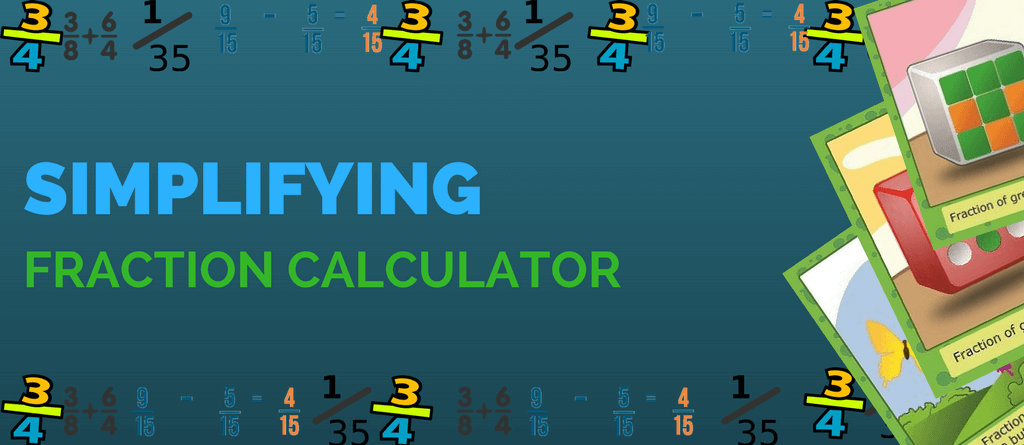Got a big scary looking fraction to solve? No need to worry, this online fraction calculator will help you in reducing/simplifying your fraction problem in no time. NowÂ simplifying fractions is as easy as solving 2 + 2 on your head. ThisÂ simplify fractions calculator helps you to simplify fractions in a jiffy. What’s the wait for? Let’s simplify!

## Simplify Fractions Calculator

Let’s try with an example.

Simplify 8/6

Solution:

 8 2
 6 2
##### Step 4: Reduced Fraction is
 4 3

Why just stop at a simple calculation? Know more about fractions and steps to simplifying them. It is as easy as deriving answers with this fractions calculator. So, learn more about simplifying fractions in an easy way.

Along with this, use

Hope the simplify fractions calculator Â helped you. Don’t forget to share with other fellow students.